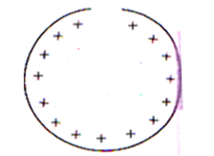Electric field
Question

# A ring of charge with radius 0.5 m has  $0.002\text{\hspace{0.17em}}\pi m$ gap. If the ring carries a charge of + 1 C, the electric field at the center is nearlyModerate
Solution

## Charge on the element opposite to the gap is$dq=\frac{Q}{2\pi r}\left(0.002\pi \right)$  $E=\frac{dq}{4\pi {\epsilon }_{0}{r}^{2}}$

Get Instant Solutions